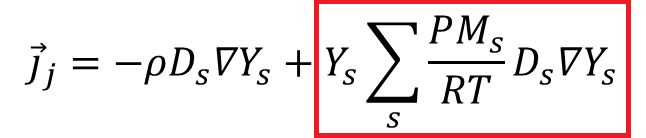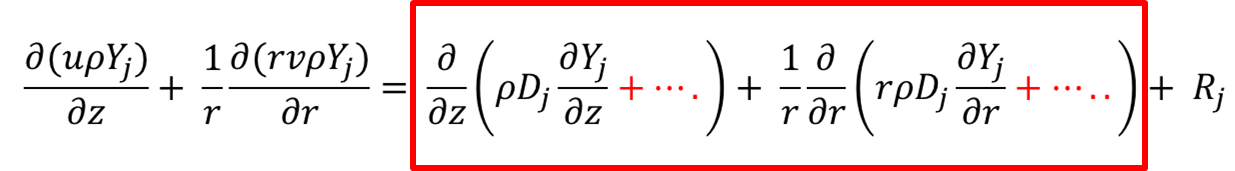Sowmiya
Subscriber

Source Terms in to mass conservation equations have the dimenion of kg/m³shttps://forum.ansys.com/discussion/comment/109994#Comment_109994

Thanks very much for the suggestionsnIf we include additional species diffusion term in the source terms of mass conservation equation having the same dimension of kg/m^3.sni.e., In my problem i need to include the terms highlighted below having unit to be kg/m.s. for four species n In case if I divide it with area (m^2) , I will get the unit to be kg/m^3.snif so by including it in source terms in mass conservation equation will it justify the inclusion of species diffusion term in species conservation equation highlighted belownPlease give me few suggestions and correct me if I am wrong.n